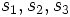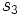# Join of subnormal subgroups is subnormal iff their commutator is subnormal

## Statement

Suppose$H,K$ are Subnormal subgroup (?)s of a group$G$. Then, the following are equivalent:

1. The join of subgroups$\langle H, K \rangle$ is a subnormal subgroup of$G$.
2. The subgroup$H^K$, i.e., the closure of$H$ under the action of$K$ by conjugation, is a subnormal subgroup of$G$.
3. The commutator of two subgroups$[H,K]$ is a subnormal subgroup of$G$.

Moreover, if$s_1, s_2, s_3$ denote the subnormal depths of the subgroups in (1),(2),(3) respectively, and$h,k$ denote the subnormal depths of$H,K$, we have:$s_2 \le s_1 + 1, s_3 \le s_2 + 1, s_3 \le s_1 + 1, s_2 \le s_3h, s_1 \le s_2k$.

## Facts used

1. Commutator of two subgroups is normal in join: For$H, K \le G$,$[H,K]$ is normal in$\langle H, K\rangle$. Further, we have$H[H,K] = H^K$,$K[H,K] = H^K$, and$KH^K = \langle H, K \rangle$.
2. Subnormality is normalizing join-closed: For$A, B \le G$ subnormal of depths$a,b$, with$B \le N_G(A)$, we have that$AB$ is subnormal of depth at most$ab$.

## Proof

Given: A group$G$ with subnormal subgroups$H, K$.

To prove: Consider the subgroups$\langle H, K \rangle$,$H^K,$, and$[H,K]$. If any one of them is subnormal, so are the others. Further, if$s_1, s_2, s_3$ are their subnormal depths, we have:$s_2 \le s_1 + 1, s_3 \le s_2 + 1, s_3 \le s_1 + 1, s_2 \le s_3h, s_1 \le s_2k$.

### Bounding the commutator in terms of the join

To prove: If$\langle H, K \rangle$ is subnormal of depth$s_1$ , so is$[H,K]$, and if$s_3$ denotes its subnormal depth, we have$s_3 \le s_1 + 1$.

Proof: By fact (1), the commutator$[H,K]$ is a normal subgroup of$\langle H, K \rangle$, and thus, if$\langle H, K \rangle$ is subnormal of depth$s_1$, we have a subnormal series for$[H,K]$ of length$s_1 + 1$. This yields$s_3 \le s_1 + 1$.

### Bounding the commutator in terms of the closure

To prove: If$H^K$ is subnormal of depth$s_2$, then$[H,K]$ is subnormal of depth$s_3$ with$s_3 \le s_2 + 1$.

Proof: By fact (1),$[H,K]$ is normal in$\langle H, K \rangle$, and in particular, is normal in the intermediate subgroup$H^K$. Thus, if$H^K$ has subnormal depth$s_2$, we obtain a subnormal series for$[H,K]$ of length$s_2 + 1$, yielding$s_3 \le s_2 + 1$.

### Bounding the closure in terms of the join

To prove: If$\langle H, K \rangle$ is subnormal of depth$s_1$, then$H^K$ is subnormal of depth$s_2$ with$s_2 \le s_1 + 1$.

Proof: We have$KH^K = \langle H, K \rangle$, and by definition,$K$ normalizes$H^K$. Thus,$H^K$ is normal in$\langle H, K \rangle$. If$\langle H, K \rangle$ is subnormal of depth$s_1$, we get a subnormal series for$H^K$ of length$s_1 + 1$, yielding$H^K$ is subnormal with subnormal depth at most$s_1 + 1$.

### Bounding the closure in terms of the commutator

To prove: If$[H,K]$ is subnormal of depth$s_3$ and$H$ is subnormal of depth$h$, then$H^K$ is subnormal of depth at most$s_3h$.

Proof: By fact (1),$H$ normalizes$[H,K]$ and$H^K = H[H,K]$. Thus, by fact (2),$H^K$ is subnormal of depth at most$s_3h$.

### Bounding the join in terms of the closure

To prove: If$H^K$ is subnormal of depth$s_2$ and$K$ is subnormal of depth$k$, then$\langle H, K \rangle$ is subnormal of depth at most$s_2k$.

Proof: We have$KH^K = \langle H, K \rangle$, with$K$ normalizing$H^K$. Thus, by fact (2),$\langle H, K \rangle$ is subnormal of depth at most$s_2k$.